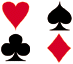# Understanding Probability

In this unit, we'll take a closer, more in-depth look at Probability Theory. Once introduced to the topic, we will take a look at experiments, outcomes, sample spaces, events, and conditional probability theory. Our lesson below provide you with interactive spinners and die rolls that are truly random. Understanding probability is the first step to being able to make predictions, determining outcomes. Use our lessons below to get started or browse other units.Probability Theory Description Introduction to Probability To begin, we will introduce probability theory through simple experiments. Yoiu will use the formula for finding the probability of an event with equally likely and non-equally likely outcomes. Certain and Impossible Events To understand the theory behind certain and impossible events. To classify experiments accordingly. To compute related probabilities. Sample Spaces The objective of this lesson is to determine the sample space of an experiment by examining each possible outcome. The Complement of an Event To define and identify the complement of an event. To find related probabilities. Mutually Exclusive Events To understand the theory behind mutually exclusive and non-mutually exclusive events. To classify an experiment accordingly. Venn diagrams and other visuals are used. Addition Rules for Probability To find the probability of mutually exclusive events by applying the addition rule. Independent Events To understand the theory behind independent events. To use the multiplication rule to compute related probabilities. Dependent Events To understand the theory behind dependent events. To use a modified version of the multiplication rule to compute related probabilities. Conditional Probability To understand the theory behind conditional probability. To derive the formula for finding conditional probabilities, and to compute related probabilities. Practice Exercises To complete 10 additional exercises as practice. To assess students' understanding of all probability theory presented. Challenge Exercises To solve 10 additional problems that challenge students' understanding of probability theory. Problems are drawn from real-life situations. To hone students' problem-solving skills. Solutions To review complete solutions to all exercises in this unit. Includes the problem, step-by-step solutions, and final answer for each exercise. Learning Objectives Introduction, certain and impossible events, sample spaces, complement, mutually exclusive events, addition rules, independent and dependent events, conditional probability.